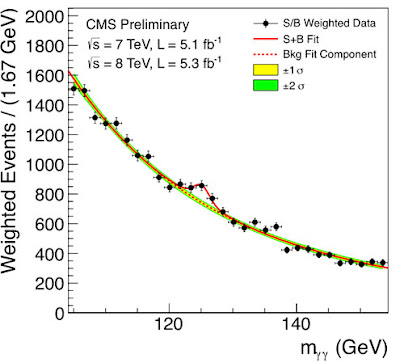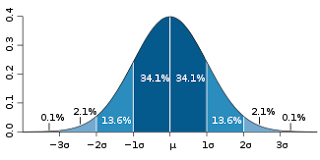## Thursday, July 05, 2012

### A guide to the Higgs boson for the perplexed

Mass and the Higgs field

The standard model of particle physics is an application of quantum field theory, and the latter holds that the fundamental structure of the physical world consists of quantum fields on space-time. Within quantum field theory, particles are represented as localised excitation states of the underlying quantum fields.

One of the fields postulated by the standard model is the Higgs field. The excitation states of the Higgs field are Higgs bosons. Whilst the Higgs field permeates all of space, Higgs bosons are localised excitations of that field, and at the energy levels available in a universe 14 billion years old, these are difficult to produce.

According to modern cosmology, the Higgs field dropped into its 'vacuum' state (i.e., its lowest energy state) when the universe was only 10-11 s old. However, the potential energy function of the Higgs field is such that its lowest energy state corresponds to a non-zero value of the Higgs field. This value is referred to as the vacuum expectation value of the Higgs field.

The Higgs field is represented to interact with all the quarks and all the leptons (e.g. electrons) in the universe. When the universe was younger than 10-11 s, the quarks and leptons were believed to be massless. Since the time at which the Higgs field dropped into its vacuum state, the non-zero vacuum expectation value of the Higgs field is considered to be responsible for the masses of the quarks and leptons.

The Higgs field is also a self-interacting field, so the Higgs field is considered to be responsible for the mass of the Higgs boson itself.

The statistics of Higgs detectionFigure 1
﻿The Higgs boson has not been directly detected at CERN. Instead, the Higgs decays into various different particles which can be detected. For example, sometimes the Higgs decays into a pair of gamma-ray photons, and sometimes it decays into a pair of Z particles. These are referred to as detection ‘channels’.

For example, Figure 1 depicts the number of gamma-gamma detection events as a function of their energy. The red dotted line plots the ‘background’, which in this context is the number of expected gamma-gamma events, as a function of energy, if the Higgs boson hadn’t been produced.

Assuming there is no Higgs production, at each energy level there is a normal (‘Gaussian’) distribution over the number of detection events (see Figure 2). This distribution has a standard deviation (‘sigma’), and by taking integer multiples of sigma, confidence bars can be plotted either side of the red dotted line in Figure 1.

This approach enables one to estimate the probability of a false positive. Thus, if the number of detection events at a particular energy is outside the 3sigma bars, it means that the probability of that result being produced by the play of chance alone is less than 0.3%. By requiring a result to be established at the 5sigma level, this means that the probability of it being a false positive is less than 0.0001%.Figure 2
In the case of Figure 1, the result from the CMS detector, there appears to be a particle which has decayed into pairs of gamma-ray photons with an energy of 125 GeV, at the 5sigma level of confidence.

A couple of other points should be noted from Figure 1. Firstly, particle physicists use the term ‘luminosity’ to refer to the flux, and 'integrated luminosity' to refer to the ‘fluence’. The latter is the total number of incident particles per unit area over the course of the experiment.

The standard unit of integrated luminosity in use at CERN is the inverse femtobarn (fb-1). A barn (b) is 10-24 cm2, and a femtobarn is 10-15 b. Thus, an integrated luminosity of 5.3 fb-1 means that there was a fluence of 5.3 particles per femtobarn of area.

The number of detected events, (in the case of Figure 1 the bump at 125 GeV), is dubbed the ‘signal strength’. Now, in general, the number of reactions per unit fluence is called the ‘cross-section’ of a reaction, and is specified in units of area. Thus, by multiplying the fluence (integrated luminosity) with the cross-section for Higgs production, the number of Higgs particle production events can be estimated.

However, only a fraction of the Higgs particles will decay into pairs of gamma-rays, and this fraction is specified by the so-called ‘branching ratio’. Thus, the number of detection events N in a particular channel will be the product of the fluence F with the Higgs production cross-section C and the branching ratio R for that channel:

N = F x C x R

Given the experimentally ascertained signal strength, and the known fluence, the quantum field theory for the Higgs field must supply a consistent Higgs production cross-section and branching ratio.

Sean said...

Peter Higgs idea was heresy when he first came up with it. Yet another scientific turnaround.

SO come on Gordon have a punt, Mr and Mrs Taxpayer have put up a lot of wonga for this, what kind of returns might we expect? Spin offs ect.

Gordon McCabe said...

I've seen an estimate that the UK's contribution costs £2 per taxpayer per year. I'd worry more about the cost of the war in Afghanistan.

Spin-offs? I hope there's none, just to irritate the commercially-minded. Having said that, I'm sure anti-gravity jet-pacs must be just around the corner.

Sean said...

Defence spending is like an insurance premium, you wonder what the point is until you need it.

I worry about corrupt ideas like aircraft carriers that need battle groups and masses of backup on land and sea to run properly, escalating costs beyond what is on paper a smallish initial cost.

And so too the Collider, its much more than £2. Its a capping stone on the pyramid, the pyramidion I believe? its the Pyramid itself that leads to the spin offs. A better question then is the collider the pyramidion or are we going higher?

I think the taxpayer can be justly proud of our contribution and returns thus far. The UK has I believe produced more Nobel winners per head of population than anywhere else. Not sure if that stat included the non science, in memory of Nobel, economic prize.

As for anti-gravity jet packs as a PPL holder, more stuff in the air would spoil things a bit for me so you can hold off on that one.